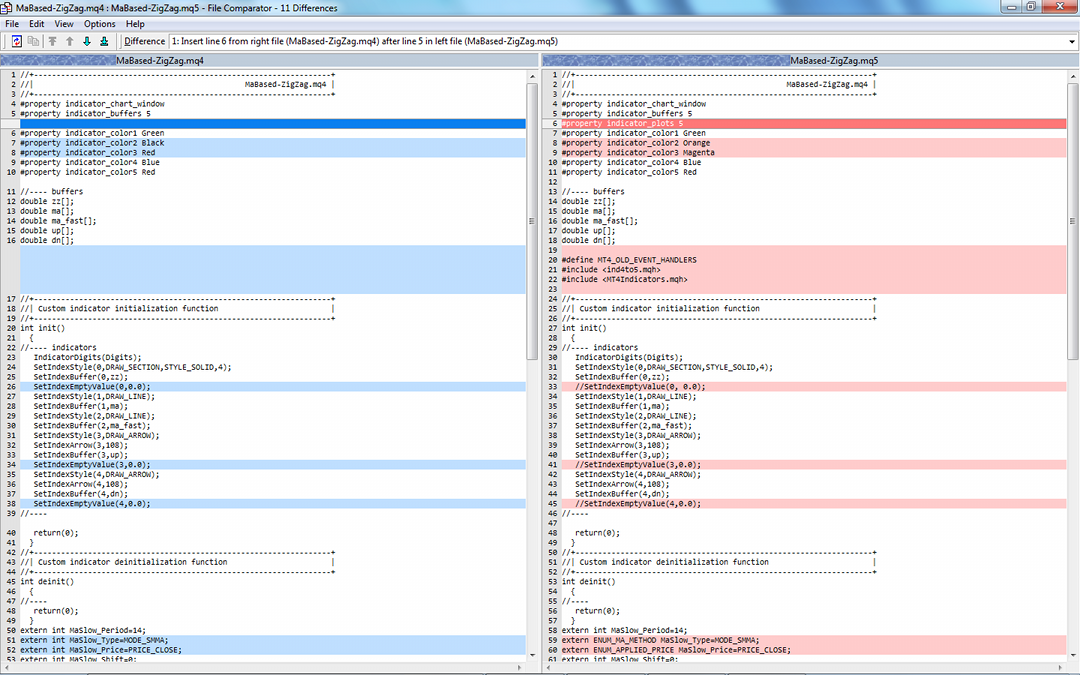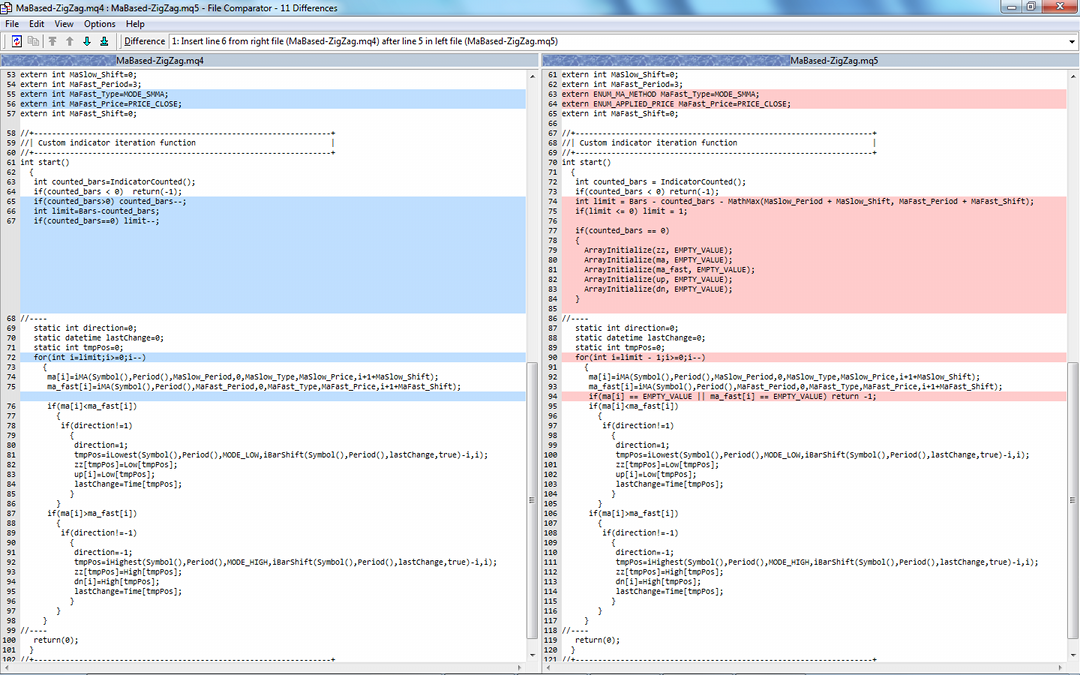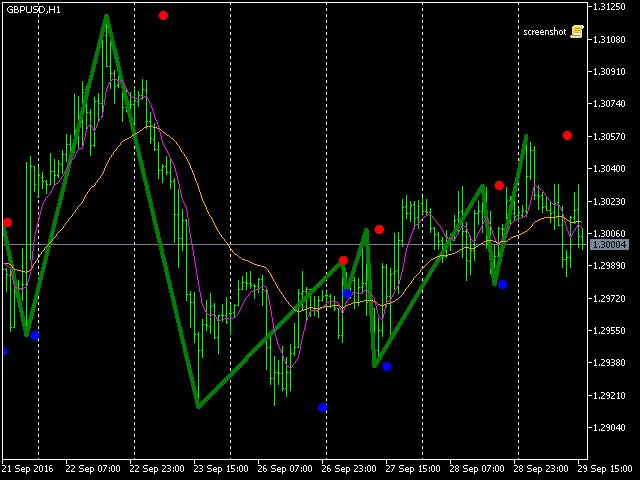MetaTrader 5 is not back compatible with MetaTrader 4 in many aspects. This is bad, but we can do nothing with this. Yet we can do something to simplify translation of MetaTrader 4 products into MetaTrader 5.

One of the problems that arises when we move from MetaTrader 4 to MetaTrader 5 is the way how latter uses different method of indicator invocation. In MetaTrader 4, a call to an indicator returns a value right away, whereas in MetaTrader 5 the similar call returns an indicator handle, which should be passed later to CopyBuffer function in order to get the value. We faced this problem in the previous blog post about converting indicators. Actually, the problem is general for any MQL program using indicators, including expert advisers. So if we solve it, we'll kill 2 birds in one shot.

We have chosen MaBased-ZigZag.mq4 (from the codebase) for example translation, and this indicator uses iMA internally (2 different instances, in fact). Let's think of a way how to emulate the old-fashined iMA behaviour in MetaTrader 5.

Because the workaround should work with all indicators we start a new class as a container and place related stuff inside.

```class Indicators
{
public:
static double jMA(const string symbol, const ENUM_TIMEFRAMES period, const int ma_period, const int ma_shift, const ENUM_MA_METHOD ma_method, const ENUM_APPLIED_PRICE applied_price, const int bar)
{
// ???
return EMPTY_VALUE;
}
```

Here we define a static function jMA which replicates iMA prototype from MetaTrader 4. When this function is called first time, we need to create an indicator and save the handle. When this function is called second and all successive times, we use the handle to call CopyBuffer and return values (if they are ready). A draft of the code could look like this.

```  private:
static int handle = -1; // this is not a MQL ;-)

static double jMA(const string symbol, const ENUM_TIMEFRAMES period, const int ma_period, const int ma_shift, const ENUM_MA_METHOD ma_method, const ENUM_APPLIED_PRICE applied_price, const int bar)
{
if(handle == -1)
{
handle = iMA(symbol, period, ma_period, ma_shift, ma_method, applied_price);
}
else
{
double result;
if(CopyBuffer(handle, 0, bar, 1, result) == 1)
{
return result;
}
}
return EMPTY_VALUE;
}
```

But wait - there may exist many different instances of the indicator, every one with its own set of parameters. So instead of the single handle variable we need a kind of array which saves not only the handles but the sets of corresponding parameters. In other words, we need an associative array with elements which can be addressed by a composite index containing all parameters. For that purpose a hashmap can be used. The name comes from the fact that indices are usually calculated as a hash of the data, but this is not a requirement.

Lets put the indicator aside for a while and concentrate on the hashmap. It should be a general purpose tool, so let use template with types placeholders.

```template<typename K, typename V>
class HashMapSimple
{
private:
K keys[];
V values[];
int count;
K emptyKey;
V emptyValue;

public:
HashMapSimple(): count(0), emptyKey(NULL), emptyValue((V)EMPTY_VALUE){};
HashMapSimple(const K nokey, const V empty): count(0), emptyKey(nokey), emptyValue(empty){};
```

Keys and values can be of a different type, this is why there are 2 arrays for underlying data (just to clarify - there is a single type for keys, and single type for values, but these 2 types can differ). For both arrays we need to provide constants denoting empty values.

Further implementation is straightforward. Here are methods for adding and retrieving data.

```    void add(const K key, const V value)
{
ArrayResize(keys, count + 1);
ArrayResize(values, count + 1);
keys[count] = key;
values[count] = value;
count++;
}
```

Get index by key:

```    int getIndex(K key) const
{
for(int i = 0; i < count; i++)
{
if(keys[i] == key) return(i);
}
return -1;
}
```

Get key by index:

```    K getKey(int index) const
{
if(index < 0 || index >= count)
{
Print(__FUNCSIG__, ": index out of bounds = ", index);
return NULL;
}
return(keys[index]);
}
```

Get value by index (as in conventional arrays):

```    V operator[](int index) const
{
if(index < 0 || index >= count)
{
Print(__FUNCSIG__, ": index out of bounds = ", index);
return(emptyValue);
}
return(values[index]);
}
```

Get value by key (associative array feature):

```    V operator[](K key) const
{
for(int i = 0; i < count; i++)
{
if(keys[i] == key) return(values[i]);
}
Print(__FUNCSIG__, ": no key=", key);
return(emptyValue);
}
```

Also we provide methods to update and remove (key;value) pairs.

```    void set(const K key, const V value)
{
int index = getIndex(key);
if(index != -1)
{
values[index] = value;
}
else
{
add(key, value);
}
}
```

Removal does actually mark an element as removed and does not resize the arrays. This allows you to remove many elements in a row, before the arrays will be shrinked by purge method shown below.

```    void remove(const K key)
{
int index = getIndex(key);
if(index != -1)
{
keys[index] = emptyKey;
values[index] = emptyValue;
}
}
```

Purge wipes out elements marked as removed and squeezes the arrays:

```    void purge()
{
int write = 0;
int i = 0;
while(i < count)
{
while(keys[i] == emptyKey)
{
i++;
}

while(keys[i] != emptyKey)
{
if(write < i)
{
values[write] = values[i];
keys[write] = keys[i];
}
i++;
write++;
}
}
count = write;
ArrayResize(keys, count);
ArrayResize(values, count);
}
```

All this is not optimized in any way but sufficient for our purpose. Types should be standard MQL types, not classes. Also type of keys can not be int because int is already used for accessing elements by indices. If necessary though, one may use long or uint for keys as a replacement.

Having this class, we can declare the following hashmap, for example:

`HashMapSimple<datetime,double> map;`

The resulting header file HashMapSimple.mqh is attached below.

Now let's return back to the indicator class and add a map into it.

```#include <HashMapSimple.mqh>

class Indicators
{
private:
static HashMapSimple<string,int> mapOfInstances;
```

In our simple implementation we'll use string keys. As the map is static it's used for all instances of MA indicators and even can be used for different types of indicators (such as WPR, ATR, etc). For that purpose we should include indicator name into the key along with parameters. The values are int handles.

We need to initialize the map object after the class definition:

`HashMapSimple<string,int> Indicators::mapOfInstances("", NO_VALUE);`

The NO_VALUE is defined as -1:

`#define NO_VALUE -1`

This is invalid handle.

Here is how MA can be coded now:

```    static double jMA(const string symbol, const ENUM_TIMEFRAMES period, const int ma_period, const int ma_shift, const ENUM_MA_METHOD ma_method, const ENUM_APPLIED_PRICE applied_price, const int bar)
{
string key = StringFormat("iMA-%s-%d-%d-%d-%d-%d", symbol, period, ma_period, ma_shift, ma_method, applied_price);
int handle = mapOfInstances[key];
if(handle == NO_VALUE)
{
handle = iMA(symbol, period, ma_period, ma_shift, ma_method, applied_price);
if(handle != INVALID_HANDLE)
{
mapOfInstances.add(key, handle);
}
}
else
{
double result;
if(CopyBuffer(handle, 0, bar, 1, result) == 1)
{
return result;
}
}
return EMPTY_VALUE;
}
```

Please notice how the key is constructed from "iMA" name, symbol, period and other input parameters. Other indicators can be implemented in the similar way, but as an additional demonstration of the idea let us support iCustom function.

iCustom is problematic because it contains an arbitrary list of parameters - their types and number can change. The only solution is to provide several variants of iCustom - one for every specific number of parameters (up to some reasonably large number) and utilize templates for type changes. For example, iCustom for 2 parameters is as follows:

```    template<typename T1, typename T2>
static double jCustom(const string symbol, const ENUM_TIMEFRAMES period, const string name, T1 t1, T2 t2, const int buffer, const int bar)
{
string key = StringFormat("iCustom-%s-%d-%s-%s-%s", symbol, period, name, (string)t1, (string)t2);
int index = mapOfInstances[key];
if(index == NO_VALUE)
{
int handle = iCustom(symbol, period, name, t1, t2);
if(handle != INVALID_HANDLE)
{
mapOfInstances.add(key, handle);
}
}
else
{
double result;
if(CopyBuffer(index, buffer, bar, 1, result) == 1)
{
return result;
}
}
return EMPTY_VALUE;
}
```

The key comprises "iCustom", symbol, period, custom indicator name, and 2 templatized parameters. For, say, 5 parameters we need similar but more lengthier implemenation:

```    template<typename T1, typename T2, typename T3, typename T4, typename T5>
static double jCustom(const string symbol, const ENUM_TIMEFRAMES period, const string name, T1 t1, T2 t2, T3 t3, T4 t4, T5 t5, const int buffer, const int bar)
{
string key = StringFormat("iCustom-%s-%d-%s-%s-%s-%s-%s-%s", symbol, period, name, (string)t1, (string)t2, (string)t3, (string)t4, (string)t5);
int index = mapOfInstances[key];
if(index == NO_VALUE)
{
int handle = iCustom(symbol, period, name, t1, t2, t3, t4, t5);
if(handle != INVALID_HANDLE)
{
mapOfInstances.add(key, handle);
}
}
else
{
double result;
if(CopyBuffer(index, buffer, bar, 1, result) == 1)
{
return result;
}
}
return EMPTY_VALUE;
}
```

To tie up this methods with calls of standard iMA or iCustom use simple defines:

```#define iMA Indicators::jMA
#define iCustom Indicators::jCustom
```

Finally, we can return to the custom indicator MaBased-ZigZag.mq4 and finish its adaptation to MetaTrader 5. Actually, it's sufficient to include a header file with new classes discussed above. Here is the side-by-side comparison of 2 files.As you may see some changes are related to the transformation, while the others not.

For the convertion we added

`#property indicator_plots 5`

and then

```#define MT4_OLD_EVENT_HANDLERS
#include <ind4to5.mqh>
#include <MT4Indicators.mqh>
```

MT4Indicators.mqh is attached. ind4to5.mqh was published in the previous post.

The define MT4_OLD_EVENT_HANDLERS is used because the indicator uses old event handlers (init, start). We need to emulate IndicatorCounted in this case, and this is done by the macro, which acquires correct number from special OnCalculate placeholder (you may consult with ind4to5.mqh source code).

One important thing to note is that our new iMA implementation returns EMPTY_VALUE until the plot is calculated. This is a side effect of the fact that MetaTrader 5 builds indicators asynchronously. As a result, we need to skip all ticks while our iMA value is unavailable and return -1 from start (in old event handlers 0 means success, and other values denote errors). Consequently the event wrapper OnCalculate in ind4to5.mqh will return 0, and recalculation will continue until iMA is ready. To achieve this behaviour, we added the line

```if(ma[i] == EMPTY_VALUE || ma_fast[i] == EMPTY_VALUE) return -1;
```

in the main loop of the indicator.

Other changes are just fixes for problems in the original indicator:

• Colors are changed for better visibility (for example, black line is invisible on black background used for charts by default).
• Empty values of the buffers should be left EMPTY_VALUE because otherwise (when set to 0) the picture is screwed up.
• Input parameters with MA types and prices should use special enumerations ENUM_MA_METHOD and ENUM_APPLIED_PRICE.
• Limit of bar to process was incorrect.
• Buffers must be filled with empty values explicitly in MetaTrader 5.

P.S. There is still one more bug in the source code of the original indicator, but it's not marked in the comparison screenshots. You may try to figure it out. This should be easy because MetaTrader 5 will show the error dynamically, if it'll occur. I hope you'll be able to fix it yourself - homework, so to speak ;-).

After all the changes and successfull compilation we can at last see how the indicator works in MetaTrader 5.Table of contents

Files:
Share it with friends: